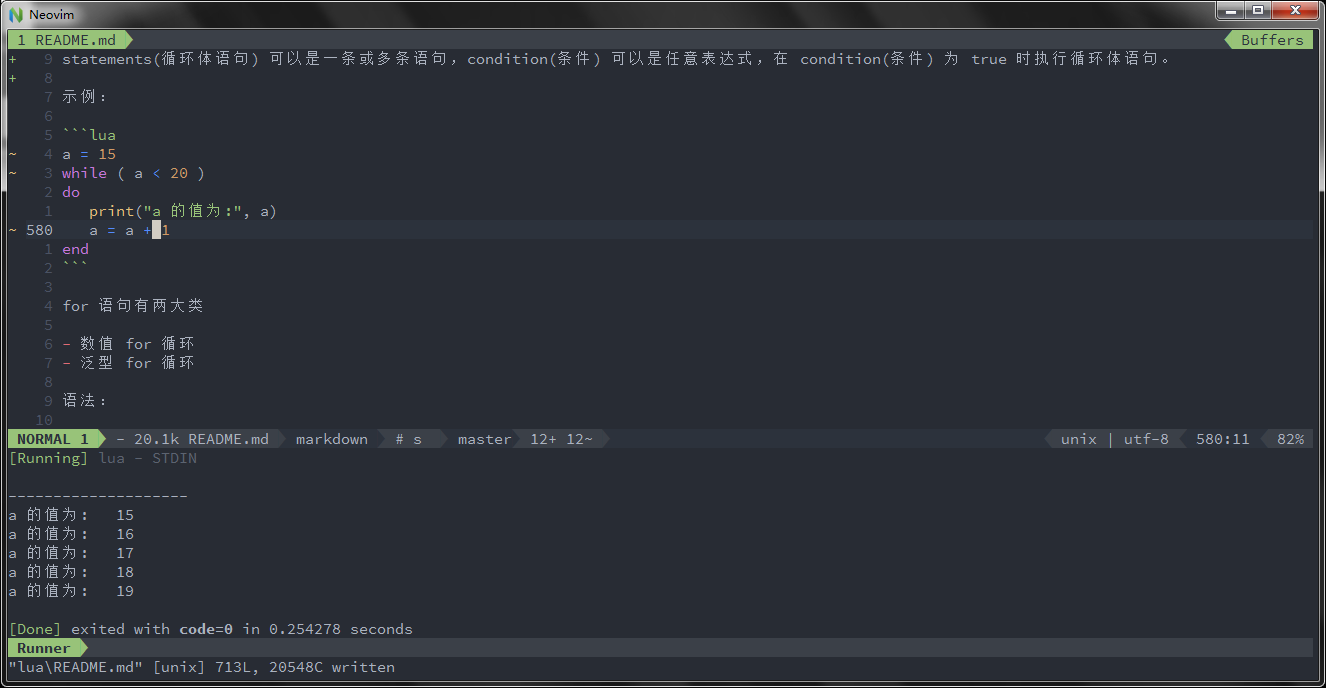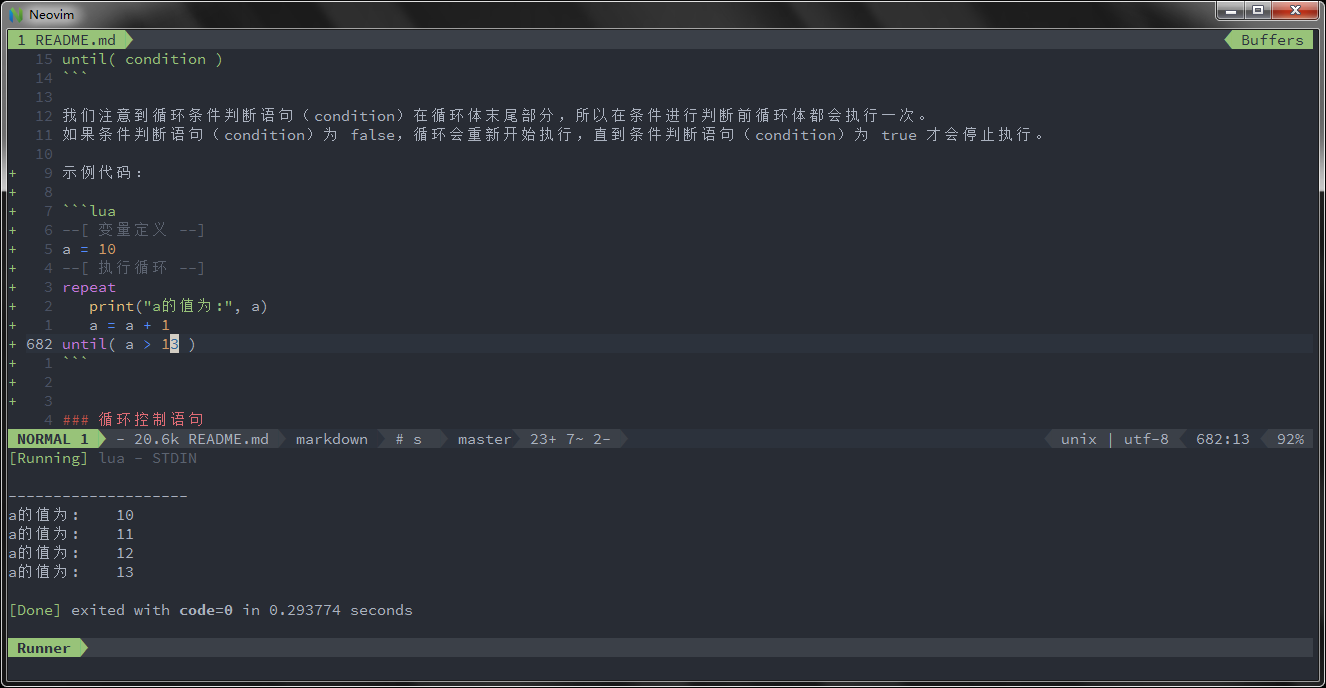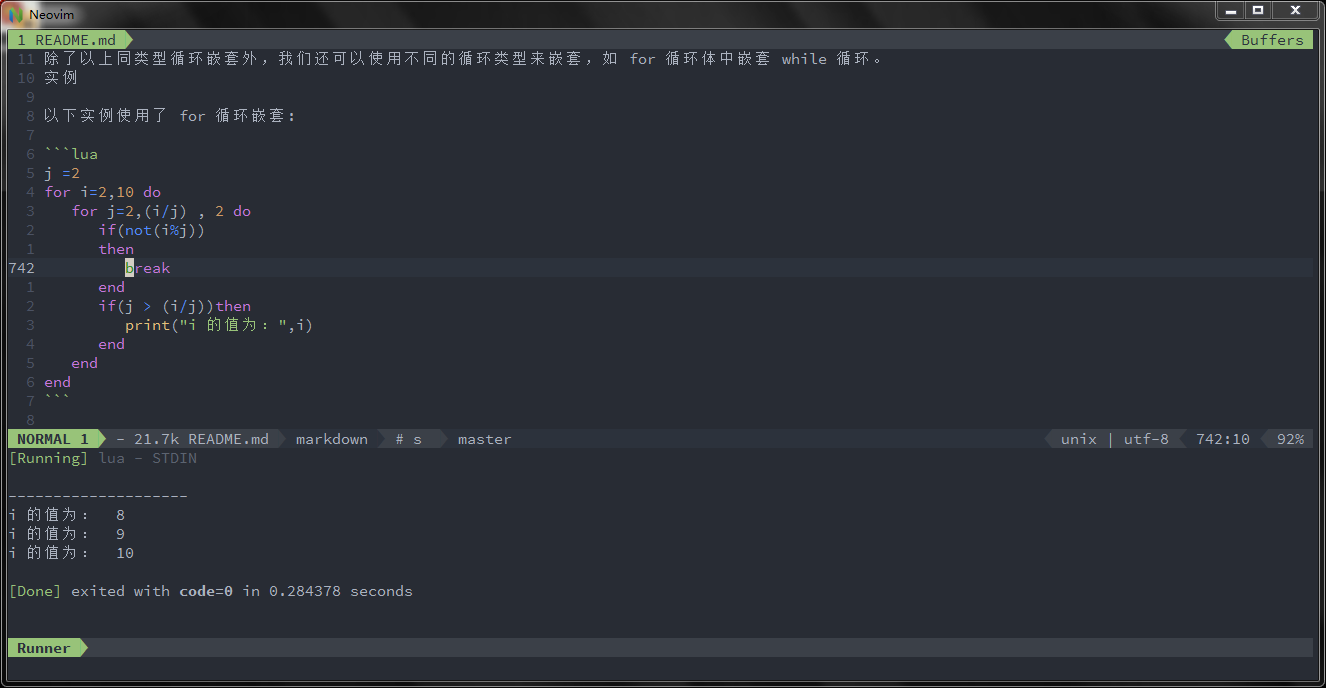My Life 时光荏苒，岁月如梭

# Lua 循环

2008-03-09

Lua 语言提供了以下几种循环处理方式：

while 循环 在条件为 true 时，让程序重复地执行某些语句。执行语句前会先检查条件是否为 true。
for 循环 重复执行指定语句，重复次数可在 for 语句中控制。
Lua repeat…until 重复执行循环，直到 指定的条件为真时为止

## while 循环

``````while(condition)
do
statements
end
``````

statements(循环体语句) 可以是一条或多条语句，condition(条件) 可以是任意表达式，在 condition(条件) 为 true 时执行循环体语句。

``````a = 15
while ( a < 20 )
do
print("a 的值为:", a)
a = a + 1
end
``````## for 循环

Lua 编程语言中 for 循环语句可以重复执行指定语句，重复次数可在 for 语句中控制。

Lua 编程语言中 for 语句有两大类：：

• 数值 for 循环
• 泛型 for 循环

### 数值 for 循环

``````for var=exp1,exp2,exp3 do
<执行体>
end
``````

var 从 exp1 变化到 exp2，每次变化以 exp3 为步长递增 var，并执行一次”执行体”。exp3 是可选的，如果不指定，默认为 1。

``````for i=1,f(x) do
print(i)
end

for i=10,1,-1 do
print(i)
end
``````

for 的三个表达式在循环开始前一次性求值，以后不再进行求值。比如上面的 f(x)只会在循环开始前执行一次，其结果用在后面的循环中。

``````function f(x)
print("方法被执行")
return x*2
end
for i=1,f(5) do print(i)
end
``````

#### 泛型 for 循环

Lua 编程语言中泛型 for 循环语法格式:

``````--打印数组a的所有值
for i,v in ipairs(a)
do print(v)
end
``````

``````days = {"Suanday","Monday","Tuesday"}
for i,v in ipairs(days) do  print(v) end
``````

## repeat until 循环

Lua 编程语言中 repeat…until 循环语句不同于 for 和 while 循环，for 和 while 循环的 条件语句在当前循环执行开始时判断，而 repeat…until 循环的条件语句在当前循环结束后判断。

``````repeat
statements
until( condition )
``````

``````--[ 变量定义 --]
a = 10
--[ 执行循环 --]
repeat
print("a的值为:", a)
a = a + 1
until( a > 13 )
``````## 嵌套循环

Lua 编程语言中允许循环中嵌入循环。以下实例演示了 Lua 循环嵌套的应用。 语法

Lua 编程语言中 for 循环嵌套语法格式:

``````for init,max/min value, increment
do
for init,max/min value, increment
do
statements
end
statements
end
``````

Lua 编程语言中 while 循环嵌套语法格式:

``````while(condition)
do
while(condition)
do
statements
end
statements
end
``````

Lua 编程语言中 repeat…until 循环嵌套语法格式:

``````repeat
statements
repeat
statements
until( condition )
until( condition )
``````

``````j =2
for i=2,10 do
for j=2,(i/j) , 2 do
if(not(i%j))
then
break
end
if(j > (i/j))then
print("i 的值为：",i)
end
end
end
``````## 循环控制语句

lua 支持 break 语句，退出当前循环或语句，并开始脚本执行紧接着的语句:

``````--[ 定义变量 --]
a = 10

--[ while 循环 --]
while ( a < 20 )
do
print("a 的值为:", a)
a = a + 1
if ( a > 13)
then
--[ 使用 break 语句终止循环 --]
break
end
end
``````

Share on:

## 评论

暂无评论

使用 GitHub issue 参与评论。

目录